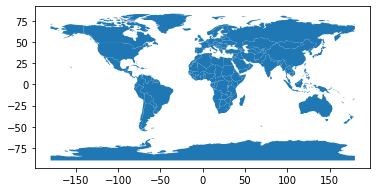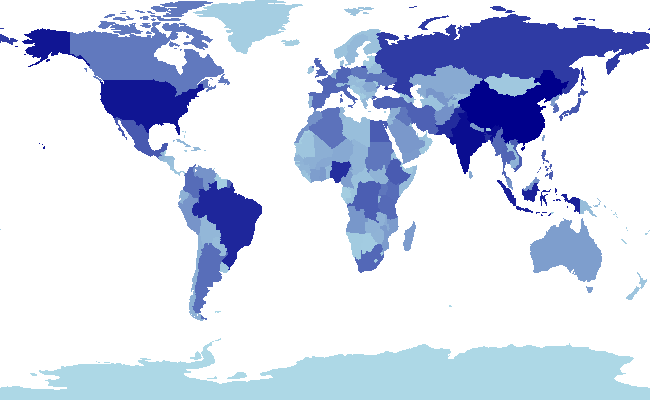# spatialpandas¶

Pandas and Dask extensions for vectorized spatial and geometric operations using numba, initially focused on the needs of data visualization libraries.

## Goal¶

The goal of spatialpandas is to provide a foundation for implementing custom vectorized geometric algorithms using numba or cython. The initial motivation for the project was to support data visualization libraries. Datashader, for example, builds on spatialpandas to support vectorized polygon rendering using numba.

## Comparison to geopandas¶

This project shares some of the goals (and some of the API) of the geopandas project. However, unlike geopandas, spatialpandas is not focused on geographic functionality. Instead, this project is focused on geometric use-cases and is explicitly independent of what space these spatial data structures and operations cover. As such, spatialpandas has no dependencies on the traditional geographic stack (GDAL, fiona, GEOS, etc.). Instead, it is built solely on top of general-purpose SciPy and PyData libraries and tools, which means that is should be easily installable using Conda or pip without any trickly binary-compatibility issues.

All algorithms are implemented in pure Python, which is then compiled to machine code at runtime with Numba. To support scaling to large problems, operations can run distributed and/or out of core using Dask.

## Future direction¶

In the future, this library could grow to support most of the geometric operations offered by geopandas. It could also be extended to include 3-dimensional geometric primitives and operations.

## Geometry Extension Arrays¶

spatialpandas provides pandas extension arrays for storing arrays of geometry objects as DataFrame columns. Unlike geopandas, where a geometry column may contain a mix of geometry types, all elements of a spatialpandas geometry array must be of the same geometry type.

In :
from spatialpandas.geometry import (
PointArray, MultiPointArray, LineArray,
MultiLineArray, PolygonArray, MultiPolygonArray
)
%matplotlib inline


### PointArray¶

The PointArray stores the coordinates of a single point per element. A PointArray can be constructed from several data structures:

A 2D list or array with two columns, the first column containing the x coordinates and the second the y coordinates.

In :
point_array = PointArray([
[1, 2],
[3, 4],
[5, 6],
])
point_array

Out:
<PointArray>
[Point([1, 2]), Point([3, 4]), Point([5, 6])]
Length: 3, dtype: point[int64]

A 1D list or array of the interleaved x and y coordinates of each point

In :
point_array = PointArray([
1, 2,
3, 4,
5, 6,
])
point_array

Out:
<PointArray>
[Point([1, 2]), Point([3, 4]), Point([5, 6])]
Length: 3, dtype: point[int64]

A tuple of two 1D lists or arrays, the first containing the x coordinates and the second the y coordinates.

In :
point_array = PointArray(
([1, 3, 5], [2, 4, 6])
)
point_array

Out:
<PointArray>
[Point([1, 2]), Point([3, 4]), Point([5, 6])]
Length: 3, dtype: point[int64]

The x and y coordinates of a PointArray can be accessed using the x and y properties.

In :
point_array.x

Out:
array([1., 3., 5.])
In :
point_array.y

Out:
array([2., 4., 6.])

All coordinates can be accessed as a single array of interleaved x and y coordinates using the flat_values property.

In :
point_array.flat_values

Out:
array([1, 2, 3, 4, 5, 6])

### MultiPointArray¶

A MultiPointArray stores the coordinates of zero or more points per element. A MultiPointArray is constructed from a list of lists, where the inner lists contain the interleaved x and y coordinates of the points in that element.

In :
multipoint_array = MultiPointArray([
[1, 2, 3, 4, 5, 6],
[7, 8, 9, 10],
])
multipoint_array

Out:
<MultiPointArray>
[MultiPoint([1, 2, 3, 4, 5, 6]), MultiPoint([7, 8, 9, 10])]
Length: 2, dtype: multipoint[int64]

All of the coordinates can be accessed as a single flat array of interleaved x and y coordinates using the buffer_values property.

In :
multipoint_array.buffer_values

Out:
array([ 1,  2,  3,  4,  5,  6,  7,  8,  9, 10])

A length one tuple with an array of indices into buffer_values that separate the coordinates of individual elements is available as the buffer_offsets property.

In :
multipoint_array.buffer_offsets

Out:
(array([ 0,  6, 10], dtype=uint32),)

### LineArray¶

A LineArray stores the coordinates of one line per element. Like the MultiPointArray, a LineArray is constructed from a list of lists, where the inner lists contain the interleaved x and y coordinates of the vertices of the line in that element.

In :
line_array = LineArray([
[1, 2, 3, 4, 5, 6],
[7, 8, 9, 10],
])
line_array

Out:
<LineArray>
[Line([1, 2, 3, 4, 5, 6]), Line([7, 8, 9, 10])]
Length: 2, dtype: line[int64]

### MultiLineArray¶

A MultiLineArray stores the coordinates of zero or more lines per element. A MultiLineArray is constructed from a doubly nested list of the interleaved x and y coordinates.

In :
multiline_array = MultiLineArray([
[[1, 2, 3, 4, 5, 6], [7, 8, 9, 10]],
[[11, 12]]
])
multiline_array

Out:
<MultiLineArray>
[MultiLine([[1, 2, 3, 4, 5, 6], [7, 8, 9, 10]]), MultiLine([[11, 12]])]
Length: 2, dtype: multiline[int64]

All of the coordinates can be accessed as a single flat array of interleaved x and y coordinates using the buffer_values property.

In :
multiline_array.buffer_values

Out:
array([ 1,  2,  3,  4,  5,  6,  7,  8,  9, 10, 11, 12])

A tuple of arrays of indices into buffer_values that separate the coordinates of individual lines and multi-line elements are available as the buffer_offsets property.

In :
multiline_array.buffer_offsets

Out:
(array([0, 2, 3], dtype=uint32), array([ 0,  6, 10, 12], dtype=uint32))

### PolygonArray¶

A PolygonArray stores the coordinates of one polygon (with zero or more holes) per element. Like the MultiLineArray, a PolygonArray is constructed from a doubly nested list of interleaved x and y coordinates. Each inner list contains the interleaved x and y coordinates of a closed ring. The first ring of each element represents the filled polygon outline and should be in a counter-clockwise order. The following rings, if any, represent holes in the polygon and should be in a clockwise order.

In :
# Square from (0, 0) to (1, 1) in CCW order
outline0 = [0, 0, 1, 0, 1, 1, 0, 1, 0, 0]

# Square from (2, 2) to (5, 5) in CCW order
outline1 = [2, 2, 5, 2, 5, 5, 2, 5, 2, 2]

# Triangle hole in CW order
hole1 = [3, 3, 4, 3, 3, 4, 3, 3]

polygon_array = PolygonArray([
[outline0],
[outline1, hole1]
])
polygon_array

Out:
<PolygonArray>
[Polygon([[0, 0, 1, 0, 1, 1, 0, 1, 0, 0]]), Polygon([[2, 2, 5, 2, 5, 5, 2, 5, 2, 2], [3, 3, 4, 3, 3, 4, 3, 3]])]
Length: 2, dtype: polygon[int64]

All of the coordinates can be accessed as a single flat array of interleaved x and y coordinates using the buffer_values property.

In :
polygon_array.buffer_values

Out:
array([0, 0, 1, 0, 1, 1, 0, 1, 0, 0, 2, 2, 5, 2, 5, 5, 2, 5, 2, 2, 3, 3,
4, 3, 3, 4, 3, 3])

A tuple of arrays of indices into buffer_values that separate the coordinates of individual rings and polygon elements is available as the buffer_offsets property.

In :
polygon_array.buffer_offsets

Out:
(array([0, 1, 3], dtype=uint32), array([ 0, 10, 20, 28], dtype=uint32))

### MultiPolygonArray¶

A MultiPolygonArray stores the coordinates of one or more multi-polygons per element, where a multi-polygon consists of zero or more polygons that may each have zero or more holes. The MultiPolygonArray adds one additional level of nesting compared to the PolygonArray.

In :
# Triangle hole in CW order
outline3 = [3, 1, 4, 1, 3, 2, 3, 1]

multipolygon_array = MultiPolygonArray([
[[outline0], [outline1, hole1]],
[[outline3]]
])
multipolygon_array

Out:
<MultiPolygonArray>
[MultiPolygon([[[0, 0, 1, 0, 1, 1, 0, 1, 0, 0]], [[2, 2, 5, 2, 5, 5, 2, 5, 2, 2], [3, 3, 4, 3, 3, 4, 3, 3]]]), MultiPolygon([[[3, 1, 4, 1, 3, 2, 3, 1]]])]
Length: 2, dtype: multipolygon[int64]
In :
multipolygon_array.buffer_values

Out:
array([0, 0, 1, 0, 1, 1, 0, 1, 0, 0, 2, 2, 5, 2, 5, 5, 2, 5, 2, 2, 3, 3,
4, 3, 3, 4, 3, 3, 3, 1, 4, 1, 3, 2, 3, 1])
In :
multipolygon_array.buffer_offsets

Out:
(array([0, 2, 3], dtype=uint32),
array([0, 1, 3, 4], dtype=uint32),
array([ 0, 10, 20, 28, 36], dtype=uint32))

## spatialpandas GeoSeries and GeoDataFrame¶

While spatialpandas geometry arrays can be stored in standard pandas Series and DataFrame objects, additional functionality is provided by the GeoSeries and GeoDataFrame subclasses. These classes are designed to implement a subset of the API of the GeoSeries and GeoDataFrame classes provided by the geopandas library.

In :
from spatialpandas import GeoSeries, GeoDataFrame

In :
geo_series = GeoSeries(multipoint_array)
geo_series

Out:
0    MultiPoint([1, 2, 3, 4, 5, 6])
1         MultiPoint([7, 8, 9, 10])
dtype: multipoint[int64]
In :
geo_df = GeoDataFrame({
'multipoint': multiline_array,
'line': line_array,
'multiline': multiline_array,
'polygon': polygon_array,
'multipolygon': multipolygon_array
})
geo_df

Out:
multipoint line multiline polygon multipolygon
0 MultiLine([[1, 2, 3, 4, 5, 6], [7, 8, 9, 10]]) Line([1, 2, 3, 4, 5, 6]) MultiLine([[1, 2, 3, 4, 5, 6], [7, 8, 9, 10]]) Polygon([[0, 0, 1, 0, 1, 1, 0, 1, 0, 0]]) MultiPolygon([[[0, 0, 1, 0, 1, 1, 0, 1, 0, 0]]...
1 MultiLine([[11, 12]]) Line([7, 8, 9, 10]) MultiLine([[11, 12]]) Polygon([[2, 2, 5, 2, 5, 5, 2, 5, 2, 2], [3, 3... MultiPolygon([[[3, 1, 4, 1, 3, 2, 3, 1]]])

## GeoPandas conversions¶

GeoSeries and GeoDataFrame objects from geopandas can be converted to spatialpandas objects by passing them to the constructor of the spatialpandas equivalent.

Here is an example of loading the naturalearth_lowres dataset using geopandas as a GeoDataFrame, and then converting it to a spatialpandas GeoDataFrame.

Note: The following examples require the geopandas, matplotlib, descartes, datashader, and holoviews packages, which are not automatically installed with spatialpandas. These can be installed using pip...

$pip install geopandas matplotlib descartes datashader holoviews or conda... $ conda install -c pyviz geopandas matplotlib descartes datashader holoviews
In :
import geopandas

geopandas.datasets.get_path('naturalearth_lowres')
)
world_gp = world_gp.sort_values('name').reset_index(drop=True)
world_gp = world_gp[['pop_est', 'continent', 'name', 'geometry']]
world_gp

Out:
pop_est continent name geometry
0 34124811 Asia Afghanistan POLYGON ((66.51861 37.36278, 67.07578 37.35614...
1 3047987 Europe Albania POLYGON ((21.02004 40.84273, 20.99999 40.58000...
2 40969443 Africa Algeria POLYGON ((-8.68440 27.39574, -8.66512 27.58948...
3 29310273 Africa Angola MULTIPOLYGON (((12.99552 -4.78110, 12.63161 -4...
4 4050 Antarctica Antarctica MULTIPOLYGON (((-48.66062 -78.04702, -48.15140...
... ... ... ... ...
172 603253 Africa W. Sahara POLYGON ((-8.66559 27.65643, -8.66512 27.58948...
173 28036829 Asia Yemen POLYGON ((52.00001 19.00000, 52.78218 17.34974...
174 15972000 Africa Zambia POLYGON ((30.74001 -8.34001, 31.15775 -8.59458...
175 13805084 Africa Zimbabwe POLYGON ((31.19141 -22.25151, 30.65987 -22.151...
176 1467152 Africa eSwatini POLYGON ((32.07167 -26.73382, 31.86806 -27.177...

177 rows × 4 columns

In :
world_df = GeoDataFrame(world_gp)
world_df

Out:
pop_est continent name geometry
0 34124811 Asia Afghanistan MultiPolygon([[[66.51860680528867, 37.36278432...
1 3047987 Europe Albania MultiPolygon([[[21.0200403174764, 40.842726955...
2 40969443 Africa Algeria MultiPolygon([[[-8.684399786809053, 27.3957441...
3 29310273 Africa Angola MultiPolygon([[[12.995517205465177, -4.7811032...
4 4050 Antarctica Antarctica MultiPolygon([[[-48.66061601418252, -78.047018...
... ... ... ... ...
172 603253 Africa W. Sahara MultiPolygon([[[-8.665589565454809, 27.6564258...
173 28036829 Asia Yemen MultiPolygon([[[52.00000980002224, 19.00000336...
174 15972000 Africa Zambia MultiPolygon([[[30.740009731422095, -8.3400059...
175 13805084 Africa Zimbabwe MultiPolygon([[[31.19140913262129, -22.2515096...
176 1467152 Africa eSwatini MultiPolygon([[[32.07166548028107, -26.7338200...

177 rows × 4 columns

A spatialpandas GeoDataFrame or GeoSeries can be converted to geopandas using the to_geopandas method.

In :
world_df.iloc[[4, 2, 0]].to_geopandas()

Out:
pop_est continent name geometry
4 4050 Antarctica Antarctica MULTIPOLYGON (((-48.66062 -78.04702, -48.66062...
2 40969443 Africa Algeria MULTIPOLYGON (((-8.68440 27.39574, -4.92334 24...
0 34124811 Asia Afghanistan MULTIPOLYGON (((66.51861 37.36278, 66.21738 37...

## Visualization¶

### Visualizing geometry elements using shapely¶

Individual elements of a spatialpandas geometry array can be converted to shapely shapes using the to_shapely method, and shapely objects automatically display themselves in the Jupyter notebook.

In :
world_df.geometry.to_shapely()

Out:

### Visualizing GeoSeries with matplotlib using geopandas¶

A spatialpandas GeoSeries can be converted to a geopandas GeoSeries using the to_geopandas method, and geopandas GeoSeries objects can be visualized with matplotlib using the plot method.

In :
world_df.geometry.to_geopandas().plot()

Out:
<matplotlib.axes._subplots.AxesSubplot at 0x7f22a53bd588>A spatialpandas GeoDataFrame can be visualized with datashader. Here is an example of visualizing a multi-polygon GeoSeries using datashader. In this case, the polygons are shaded to reflect the estimated population of each country.

In :
import datashader as ds
cvs = ds.Canvas(plot_width=650, plot_height=400)
agg = cvs.polygons(world_df, geometry='geometry', agg=ds.mean('pop_est'))By combining datashader and a HoloViews DynamicMap, spatialpandas geometry arrays can be rendered interactively.
import holoviews as hv